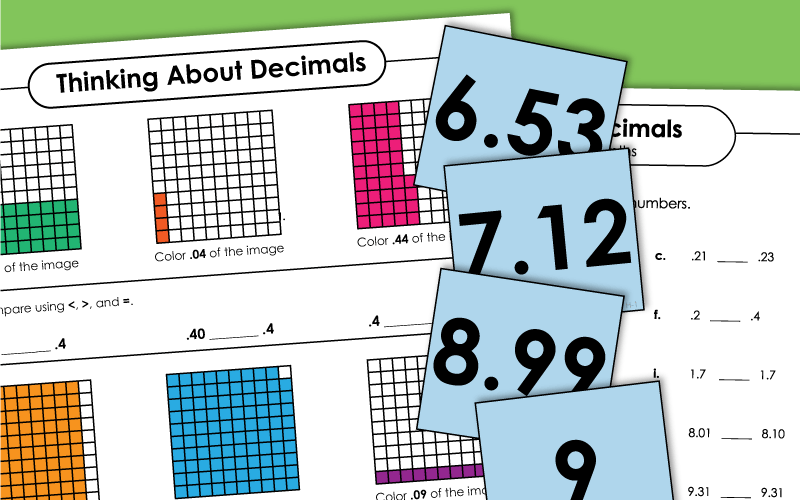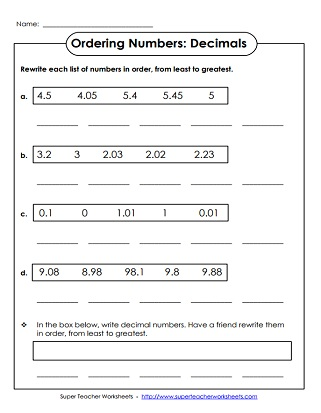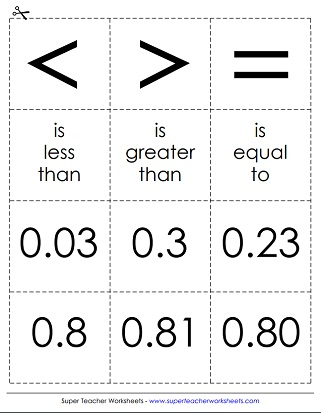# Comparing and Ordering Decimals

With these printable worksheets and card sets, your students can learn to compare and order decimal numbers.## Comparing Decimals

Use mathematical symbols (less than, equal to, greater than) to compare decimal numbers.  Decimals to tenths and hundredths.  (example:  5.8 > 5.08)
For this activity, students will use common math symbols (greater than, less than, and equal) to compare pairs of numbers with decimals in them. (example: 6.21 < 6.23)
This worksheet has three higher order thinking questions. Students order decimals, compare decimals, and ponder the answer an estimation word problem.
Use these math symbol, phrase, and number cards to help students learn to compare decimal numbers. Designed for learning centers, small group instruction, and/or classroom games; Includes tenths and hundredths.

## Ordering Decimals

Order decimal numbers from least to greatest. Each card has a number with one decimal place.
Place the cards on the floor in lines, from smallest to largest. Al numbers in this set have two decimal places.
Write each set of decimals in order, from least to greatest. Includes whole numbers, tenths, and hundredths.
These cards have numbers with one and two decimal places. Order them from smallest value to largest value.
Rearrange each set of five numbers from least to greatest. Includes decimals with tenths, hundredths, and thousandths.
Arrange the cards from least value to greatest value. The cards in this set have numbers up to thousandths.
Decimal Worksheets

Learn about basic decimals (tenths, hundredths, thousandths). Also includes place value activities and rounding decimals.

Comparing Fractions

## Worksheet ImagesMy Account
Site Information